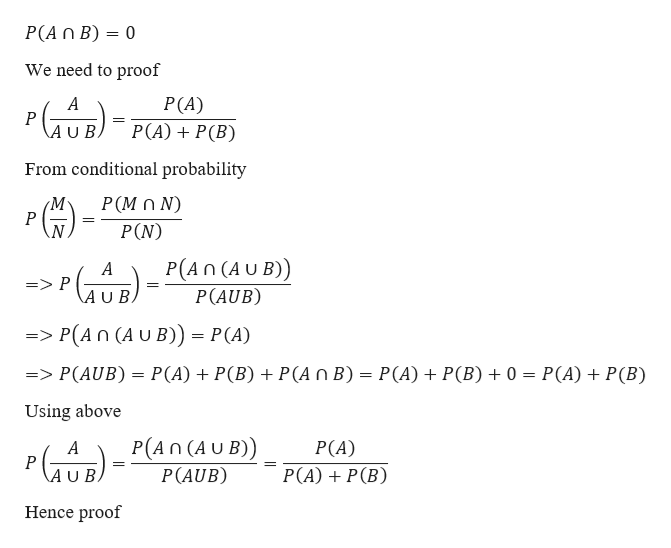# Prove that if two events, A and B, are mutually exclusive, then P(A | A U B ) = P(A) / ( P(A) + P(B) )

Question
2 views

Prove that if two events, A and B, are mutually exclusive, then P(A | A U B ) = P(A) / ( P(A) + P(B) )

check_circle

star
star
star
star
star
1 Rating
Step 1

If two events are mutually exclusive, then the two events are disjoint event.

In other words, if A a...help_outlineImage TranscriptioncloseP(An B) 0 We need to proof P(A) P(A)P(B) A P AUB From conditional probability Р(MON) P P(N) P(An (AU B)) P(AUB) A => P A U B => P(An (A U B)) P(A) P(A) + P(B) P(A n B)= P(A) + P(B) 0 = P(A) + P(B) => P(AUB) Using above P(An (A U B)) P(A) P(AUB) P(A)P(B) \A U B. Hence proof fullscreen

### Want to see the full answer?

See Solution

#### Want to see this answer and more?

Solutions are written by subject experts who are available 24/7. Questions are typically answered within 1 hour.*

See Solution
*Response times may vary by subject and question.
Tagged in

### Other